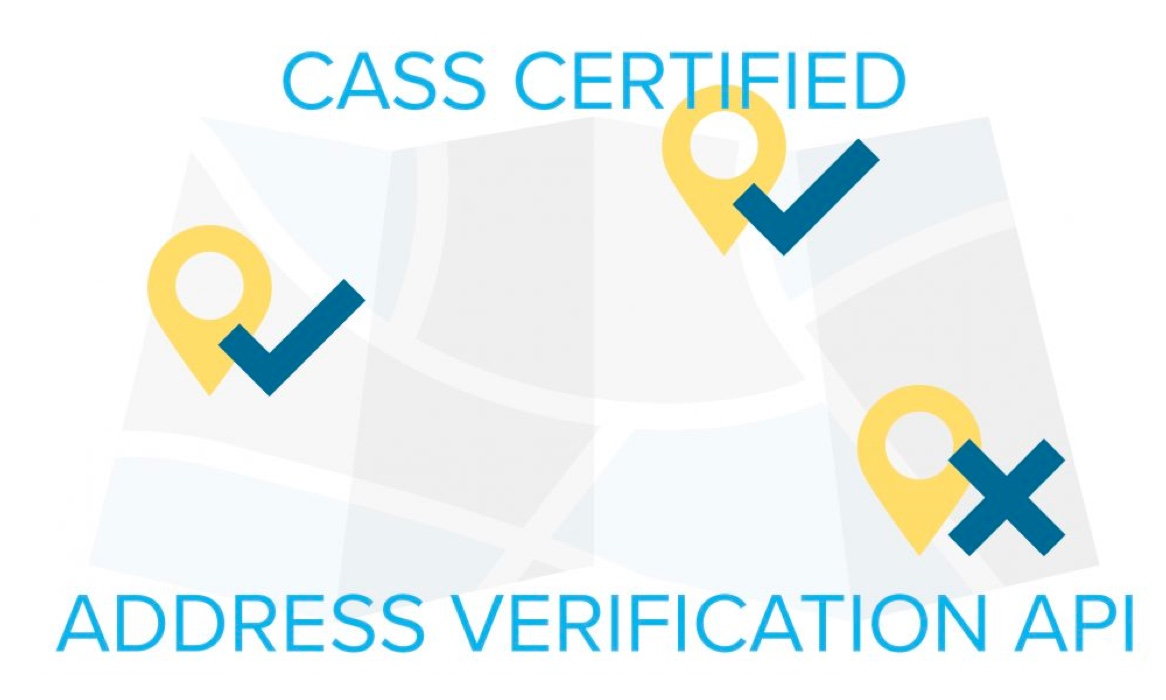### Lob’s website experience is not optimized for Internet Explorer. Please choose another browser.Lob's new Address Verification API is designed to help customers streamline their direct mail operations by maximizing the deliverability of every address before it's stored in their CRM or other data repository. This tutorial shows our new API in action. We'll build a web app in Rails that verifies an address as soon as it's entered on sign-up.

Hate to read instructions? We’ve got all the source code for this example available in a public repository.

### What are we building?

This tutorial will show you how to build a website that will determine if a mailing address is deliverable when you sign up!

### Key Tools

• Ruby on Rails – Rails is a popular web application framework running on the Ruby programming language. See installrails.com for an in-depth startup guide.
• Redis – Redis is a popular in-memory data store that we’ll be using to handle our application’s service workers. See the official quickstart guide for more info.
• Lob US Address Verification API – Lob has a whole suite of RESTful APIs for printing and mailing. We will be using the US Address Verification API in this tutorial.

Before diving into the code, we will need to register for a Lob API key.

Visit the registration page and sign up for an account. You will be given a Test API Key which will give you full access to the API in a sandbox environment. When in Test mode, the API will only return a sample address.

## Getting Started

### Generate Project

First, let’s have Rails setup up our project:

### Install Dependencies

Add the following lines to the Gemfile:

Then, install the above dependencies:

### Set Up the User Model

We’re going to be using Devise, a popular, flexible authentication solution for Rails.

After this, we’ll create a User table and model by utilizing another one of Devise’s generators.

Now that we have Devise set up, we’ll need to add a few fields to the User table to collecting a mailing address.

/db/migrate/xxxxx_add_address_to_user.rb

Now we can run the migrations.

Now, this is where the magic happens. We’ll add in a validation to check if an address is deliverable in the User model.

/models/user.rb

### Create the Sign Up Page

We’ll need to modify the registrations controller and views created by Devise to accept these relevant fields upon sign up.

/controllers/registrations_controller.rb

class RegistrationsController < Devise::RegistrationsController

/views/devise/registrations/new.html.erb

## Sign up

<%= form_for(resource,="" as:="" resource_name,="" url:="" registration_path(resource_name))="" do="" |f|="" %=""> <%= devise_error_messages!="" %=""></%=></%=>

<%= f.label="" :email="" %=""></%=>
<%= f.email_field="" :email,="" autofocus:="" true="" %=""></%=>

<%= f.label="" :password="" %=""> <% if="" @minimum_password_length="" %=""> </%></%=>(<%= @minimum_password_length="" %=""> characters minimum)</%=> <% end="" %=""></%>
<%= f.password_field="" :password,="" autocomplete:="" "off"="" %=""></%=>

<%= f.label="" :password_confirmation="" %=""></%=>
<%= f.password_field="" :password_confirmation,="" autocomplete:="" "off"="" %=""></%=>

<%= f.label="" :first_name="" %=""></%=>
<%= f.text_field="" :first_name="" %=""></%=>

<%= f.label="" :last_name="" %=""></%=>
<%= f.text_field="" :last_name="" %=""></%=>

<%= f.label="" :address_line1="" %=""></%=>
<%= f.text_field="" :address_line1="" %=""></%=>

<%= f.label="" :address_line2="" %=""></%=>
<%= f.text_field="" :address_line2="" %=""></%=>

<%= f.label="" :address_city="" %=""></%=>
<%= f.text_field="" :address_city="" %=""></%=>

<%= f.label="" :address_state="" %=""></%=>
<%= 4="" f.text_field="" :address_state,="" maxlength:="" 2,="" size:="" %=""></%=>

<%= f.label="" :address_zip="" %=""></%=>
<%= 15="" f.text_field="" :address_zip,="" maxlength:="" 11,="" size:="" %=""></%=>

<%= f.label="" :address_country="" %=""></%=>
<%= 4="" f.text_field="" :address_country,="" maxlength:="" 2,="" size:="" %=""></%=>

<%= f.submit="" "sign="" up"="" %=""></%=>

<% end="" %=""> <%= render="" "devise="" shared="" links"="" %=""></%=></%>

### Sanity Check

Let’s add a home page and some quick routing logic so we can see a preview of what we have so far. Running the command below, we’ll have a empty controller and view generated for us.

\$ rails generate controller home index

And we’ll make a very simple view.

/views/home/index.html.erb

# Homepage

You've signed up!

Now if we start up Rails, you should be able to sign up and you will see an error if you sign up with an undeliverable address.

\$ rails s

### Wrapping Up

You can see the full source code for this example here.

You can check out Lob’s documentation for more information. If you have any additional questions, don’t hesitate to leave a comment below or contact us directly. We’re always happy to help!